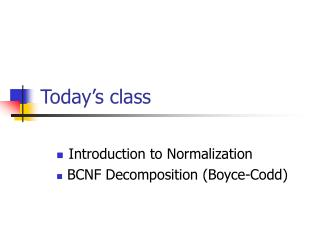# Today’s class - PowerPoint PPT PresentationDownload PresentationToday’s class

Today’s classDownload Presentation## Today’s class

- - - - - - - - - - - - - - - - - - - - - - - - - - - E N D - - - - - - - - - - - - - - - - - - - - - - - - - - -
##### Presentation Transcript

1. Today’s class Introduction to Normalization BCNF Decomposition (Boyce-Codd)

2. Normalization Consider the following Relational table ‘Movies’ Anomalies: Redundancy, Update Anomalies, Deletion Anomalies

3. Example 1 • ‘Movies’ has following schema • (Title, Year, Length, FilmType, StudioName, StarName) • Expected FD • Title Year  Length, FilmType, StudioName • { Title, Year, StarName } is the only Key • Movies relation contains ‘redundancy’ • Due to ‘multi-valued attribute’ StarName • Title, Year, Length, FilmType, StudioName repeated for every star of a movie

4. Movies1 Movies2 ‘Movies’ is divided into two tables ‘Movies1’ and ‘Movies2’ This decomposition has resulted in removal of ‘Anomalies’ It’s BCNF Decomposition

5. Loss-less Join Decomposition Condition: When R is divided into R1, R2 the decomposition is loss-less join if R1  R2  R1 or R1  R2  R2

6. BCNF • A relation R is in BCNF iff every non-trivial FD X  Y is such that X is a superkey • X should contain a key, any key • BCNF decomposition • Identify A non-trivial BCNF violating FD, X  Y • If Y = X+ fine, otherwise replace Y by X+ • Divide R into R1 with attributes X  Y and R2 with attributes X  (R-X-Y) • The above is a loss-less join decomposition • Note: Any 2-attribute relation is in BCNF

7. Back to Example 1 • ‘Movies’ has following schema • (Title, Year, Length, FilmType, StudioName, StarName) • Expected FD • Title Year  Length, FilmType, StudioName • { Title, Year, StarName } is the only Key • The FD violates BCNF condition • Doing BCNF decomposition on ‘Movies’ results in • Movies1 (Title, Year, Length, FilmType, StudioName) • Movies2 (Title, Year, StarName)

8. Example 2 : MovieStudios Given FD’s Title Year  Length, FilmType, StudioName StudioName  StudioAddr { Title, Year } is the only key Unlike Example1 all attributes here are single-valued but still redundancy exists due to ‘transitive dependency’

9. Example 3 • Let R=R(A,B,C,D,E) with FDs F={ ABC, CD, DE) • {A,B} is the only key • C  D violates the BCNF condition • C+ = {D,E} therefore consider C  DE • BCNF Decomposition • R1={C,D,E} with C  D, D  E ( {C} is the key) • R2={C,A,B} with AB  C ( {A,B} is the key) • D  E in R1 violates the BCNF • R1’={D,E} with D  E ({D} is the key) • R1’’={D,C} with C  D ({C} is the key) • Final Decomposition of R is R1’, R1’’, R2 which are all in BCNF

10. Dependency Preserving • A decomposition of R into R1 and R2 is dependency preserving if F+ = (FR1 FR2)+ where ‘FS’ (S⊆R) represents projection of F on S • Is BCNF decomposition dependency preserving? • NO

11. Example 4 • Let R=R(A,B,C) with FDs F={ BC, ACB} • Keys: {A,C}, {A,B} • BC violates BCNF condition • R1={B,C} with BC ({B} is the key) • R2={B,A} • What about ACB • BCNF decomposition is not Dependency Preserving